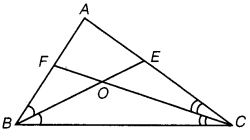NCERT Solutions for Class 9 Maths Chapter 7 Triangles Ex 7.5

# NCERT Solutions for Class 9 Maths Chapter 7 Triangles Ex 7.5

## NCERT Solutions for Class 9 Maths Chapter 7 Triangles Ex 7.5

NCERT Solutions for Class 9 Maths Chapter 7 Triangles Ex 7.5 are the part of NCERT Solutions for Class 9 Maths. Here you can find the NCERT Solutions for Chapter 7 Triangles Ex 7.5.

Ex 7.5 Class 9 Maths Question 1.
ABC is a triangle. Locate a point in the interior of ΔABC which is equidistant from all the vertices of ΔABC.

Solution:

Let OD and OE be the perpendicular bisectors of the sides BC and AC of ΔABC.
O is equidistant from two ends B and C of the line segment BC as O lies on the perpendicular bisector of BC.
Similarly, O is equidistant from C and A of the line segment AC as O lies on the perpendicular bisector of AC.

Thus, the point of intersection O of the perpendicular bisectors of sides BC, CA and AB is the required point which is equidistant from all the vertices of ΔABC.

Ex 7.5 Class 9 Maths Question 2.
In a triangle locate a point in its interior which is equidistant from all the sides of the triangle.

Solution:
Let BE and CF be the bisectors of ABC and ACB respectively intersecting AC and AB at E and F respectively.Since O lies on BE, the bisector of
ABC, hence O will be equidistant from AB and BC. Again, O lies on CF, the bisector of ACB. Hence, O will be equidistant from BC and AC. Thus, O will be equidistant from AB, BC and CA.

Ex 7.5 Class 9 Maths Question 3.
In a huge park, people are concentrated at three points (see figure)
A. where there are different slides and swings for children
B. near which a man-made lake is situated
C. which is near to a large parking and exit.

Where should an ice-cream parlor be set up so that maximum number of persons can approach it?
[Hint: The parlor should be equidistant from A, B and C.]

Solution:
The ice-cream parlor should be equidistant from A, B and C for which the point of intersection of perpendicular bisectors of AB, BC and CA should be situated.
So, O is the required point which is equidistant from A, B and C.

Ex 7.5 Class 9 Maths Question 4.
Complete the hexagonal and star shaped Rangolies [see fig. (i) and (ii)] by filling them with as many equilateral triangles of side 1 cm as you can. Count the number of triangles in each case. Which has more triangles?

Solution:
We first divide the hexagon into six equilateral triangles of side 5 cm as given below:

We take one triangle from six equilateral triangles as shown above and make as many equilateral triangles of side 1 cm as shown in the figure given below.
The number of equilateral triangles of side 1 cm = 1 + 3 + 5 + 7 + 9 = 25
So, the total number of triangles in the hexagon = 6 × 25 = 150.
To find the number of triangles in Fig. (ii), we follow the same procedure.
So, the number of triangles in Fig. (ii) = 12 × 25 = 300

Hence, Fig. (ii) has more triangles.

NCERT Solutions for Maths Class 10

NCERT Solutions for Maths Class 12